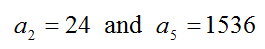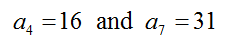# Arithmetic vs Geometric

Directions: Which is bigger?

The common ratio, r, in a geometric sequence withOR

the common difference, d, in an arithmetic sequence with### Hint

How do you find the common ratio/common difference?

Can you tell just by looking at the given information?  Why or why not?

r = 4 and d = 5, so the common difference in this case is bigger.

Source: Nanette Johnson

## Asymptotes of Rational Functions

Directions: Using the digits 1 to 9 at most one time each, place a digit …

### One comment

1.It is interesting to see the importance of subscript notation here. CCSS generally show function notation for sequences, and rightly so, because sequences are functions. However, I think it would be much messier to write this question without subscript notation.